# Electronics and Communication Engineering - Exam Questions Papers

6.

The depth of penetration at 4 MHz is 10 cm, then at 2.5 MHz it is

 A. 62.5 cm B. 13 cm C. 6.25 cm D. 8 cm

Explanation:

Depth of penetration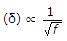δ2 = 12.64 cm.

7.

The expression given is F(A, B , C, D, E, F, G, H) =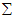(33, 48, 102, 158, 222, 229, 233, 243, 255); then the __________ and __________ minterms are grouped together into dual and variable __________ is reduced using the dual

 A. 243, 233, D B. 222, 158, B C. 243, 255, A D. 229, 243, E

Explanation:

We use Quine - McCluskey method of reduction :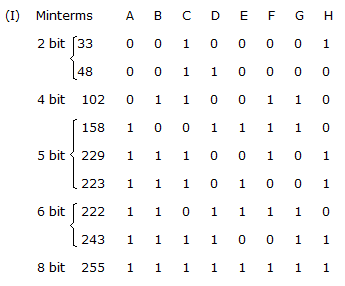We can observe that minterms 158 and 222 can be grouped together to reduce the variable 'B'.

8.

Calculate i(t) for t ≥ 0 assuming the switch has been in position A for a long time. At t = 0, the switch is moved to position B.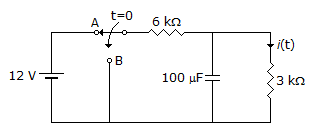A.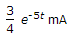B.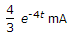C.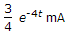D.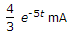Explanation: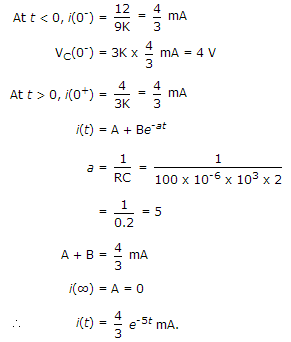9.

A source produces 4 symbols with probabilities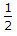,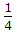,,For this source, a practical coding scheme has an average codeword length of 2 bits/symbol. The efficiency of the code is

 A. 1 B.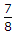C.D.Explanation:

Total information for L message is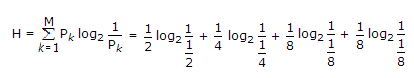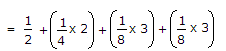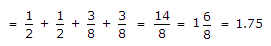Efficiency =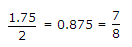.

10.

A generator of 50 Ω internal impedance and operating at 1 GHz feeds a 75 Ω load via a coaxial line of characteristic impedance 50 Ω. The VSWR on the feed line is

 A. 0.5 B. 1.5 C. 2.5 D. 1.75

Explanation:

ZL = 75 Ω

Zo = 50 Ω

Reflection coefficient: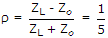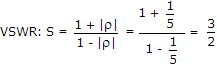.

#### Current Affairs 2022

Interview Questions and Answers